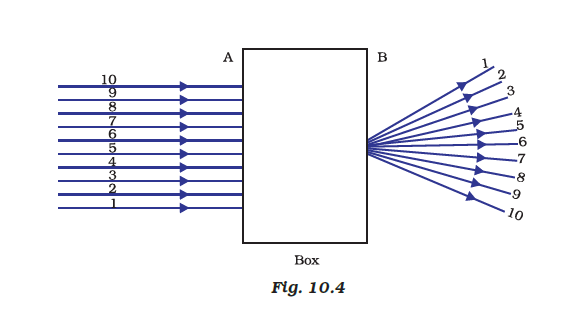# A beam of light is incident through the holes on side A and emerges out of the holes on the other face of the box as shown in Figure. Which of the following could be inside the box? (a) Concave lens (b) Rectangular glass slab (c) Prism (d) Convex lens## Convex lens

• A convex lens is a lens with an outward curve.
• The thickness at the centre of a convex lens is more than the thickness at the edges of the lens.
• Convex lenses are converging lenses. They have the ability to converge a parallel beam of light into a point.
• The lens found in the human eye is a prime example of a convex lens.
• Another common example of a convex lens is the magnifying glass that is used to correct Hypermetropia or long-sightedness.(0)(0)